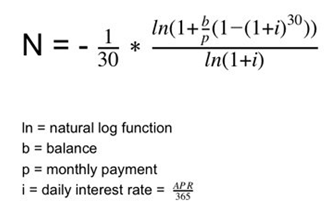# Thread: Need Help With Equation in Java

1.MemberJoin Date
Feb 2013
Posts
3
Rep Power
0

##Need Help With Equation in Java

I just started Java and this is probably a dumb question. I am trying to put the following equation into Java:In Java I typed the following (this is just a test program, my actual one has commenting/prompts):

final double FACTOR = -1/30;
double b = 0.0;
double n = 0.0;
double p = 0.0;
double dailyInterest = 0.0;
double apr = 0.0;

apr = kb.nextDouble();

b = kb.nextDouble();

p = kb.nextDouble();

dailyInterest = apr/365;

n = FACTOR*((Math.log(1+(b/p)*(1-(Math.pow(1+dailyInterest,30)))/Math.log(1+dailyInterest))));

I've tried to break it apart a few different ways and as separate variables to clean it up, but the answer I get is always "NaN" for my n value (the values I am trying to use are 13.65 for apr, 2013 for b, and 25 for p; test values provided by the professor).

Any ideas on why this would be occurring?Reply With Quote

2.MemberJoin Date
Feb 2012
Posts
59
Rep Power
0

##Re: Need Help With Equation in Java

check the value of Factor, I didn't have time to compile and test it out, but if what you wrote is exactly what you had, you may have a data type issue.

so put this somewhere:

System.out.println(FACTOR);

edit I imagine you know what i am pointing out since all of your other values are properly initialized.
Last edited by lenois; 02-08-2013 at 03:01 AM.Reply With Quote

3.MemberJoin Date
Feb 2013
Posts
3
Rep Power
0

##Re: Need Help With Equation in JavaOriginally Posted by lenoischeck the value of Factor, I didn't have time to compile and test it out, but if what you wrote is exactly what you had, you may have a data type issue.

so put this somewhere:

System.out.println(FACTOR);

edit I imagine you know what i am pointing out since all of your other values are properly initialized.
It looked like FACTOR as a fraction as outputting zero; however, even changing that to a decimal of -.013333333333, the output of my equation is still NaN...Reply With Quote

4. ##Re: Need Help With Equation in Java

If 'apr' is 'annual percentage' then i= (apr/365)/100;

kind regards,

JosReply With Quote

5.MemberJoin Date
Feb 2013
Posts
3
Rep Power
0

##Re: Need Help With Equation in JavaOriginally Posted by JosAHIf 'apr' is 'annual percentage' then i= (apr/365)/100;

kind regards,

Jos
Thank you! I did (apr/100)/365 and it seems that the equation is working now!Reply With Quote

####Posting Permissions

• You may not post new threads
• You may not post replies
• You may not post attachments
• You may not edit your posts
•